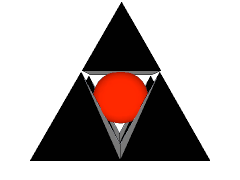Ontario Association for Mathematics Education
Ontario Association for Mathematics Education

### Ontario Mathematics Olympiad

#### Sample Question #3

NOTE: This was an event for two students.

#### The Missing Number

Many ancient civilizations have contributed to our mathematical knowledge. In Babylonia, Egypt, China, and India, as well as in the Greek, Roman, and Islamic Empires, the study of mathematics was highly valued, and all these civilizations made significant contributions to various areas of mathematics, including geometry, arithmetic, and algebra.

Many mathematicians have worked out interesting puzzles involving computations. Here is an example for you to work on:

Write down any two numbers that use all the digits from 0 to 9 exactly once. For example, you could write:

342195 and 6087

Next, add the two numbers. Finally, erase the original two numbers and any digit in the answer.

 342195 >>> 34 ? 282 +  6087 348282

They understood that you could glance at the number 34 ? 282 and immediately say, "The missing number is 8".

Can you supply the missing digit in the following, and explain how it is possible to guess the missing digit in the answer so quickly?

1 ? 1341

Try to find the missing digit in the following numbers:

 5 ? 3086 54 ? 028 ? 31346 10899 ?